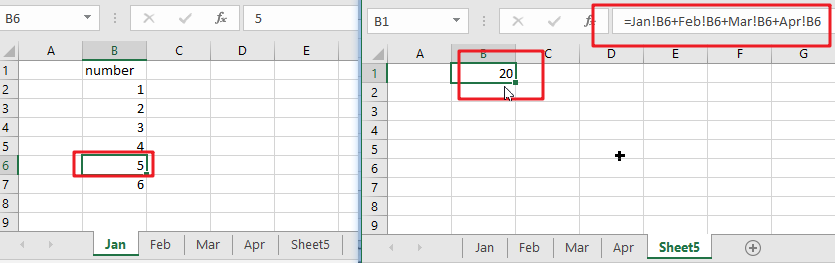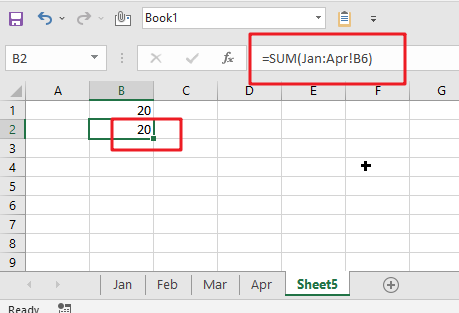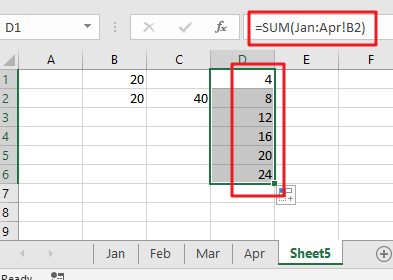# How to Use 3D SUM Multiple Worksheets

To sum a range of numbers is straightforward for most Excel users, but do you know how to establish a 3D reference to total the same range of numerous sheets. In this post, I will present the steps for this work. Let’s explore formulae to construct a 3D sum multiple worksheets in Excel.

## 3D SUM Multiple Worksheets in Microsoft Excel

The primary objective of this formula is to sum the same range in one or more sheets. Here we will learn how to construct a 3D SUM numerous spreadsheets in Microsoft Excel. That means, with the assistance of a formula based on the SUM function with the 3D reference you may able to sum the same range on one or more sheets. So, with the aid of this formula, you may able to build a 3D SUM numerous spreadsheets in Microsoft Excel.

## What is a 3D reference in Excel?

As indicated above, an Excel 3D reference enables you refer to the same cell or a range of cells in numerous spreadsheets. In other words, it addresses not just a range of cells, but also a range of worksheet names. The crucial thing is that all of the referred sheets should have the same pattern and the same data type. Please examine the following example.

Supposing you have monthly sales data on 4 separate sheets:

What you are seeking for is figuring out the grand total, i.e., adding up the sub-totals in four monthly sheets. The most apparent approach that comes to mind is sum up the sub-total cells from all the worksheets in the normal way:

`=Jan!B6+Feb! B6+Mar!B6+Apr!B6`However, what if you have 12 sheets for a whole year, or even many years’ worth of sheets? This would entail a significant amount of labor. Alternatively, you may use the SUM function in conjunction with a three-dimensional reference to sum across sheets:

`=SUM(Jan:Apr!B6)`This SUM formula does the same computations as the longer formula above, that is, it sums the values in cell B6 in all the sheets between the two border worksheets specified, in this case Jan and Apr.

Notes:

If you want to duplicate your three-dimensional formula to several cells and do not want the cell references to change, you may secure them by include the \$ symbol, i.e. by using absolute cell references such as:

` =SUM(Jan:Apr!\$B\$6)`

You are not even need to compute a sub-total in each monthly sheet; just mention the range of cells to be calculated in your 3D formula:

`=SUM(Jan:Apr!B2:B5)`

## Calculating a range of cells on many sheets using a 3D formula in Excel

If you wish to know the total sales for each particular product, create a summary table in which the items appear precisely as they do on monthly sheets, then enter the following three-dimensional formula in the top-most cell, B2 in this example:

=SUM(Jan:Apr!B2)Use a relative cell reference without the \$ symbol to ensure that the formula is modified for additional cells when copied along the column.

Let’s create a generic Excel 3D reference and formula based on the examples above.

### 3D SUM Multiple Worksheets General Formula:

`=SUM(Sheet1:Sheet3!A1)`

### Related Functions

• Excel SUM function
The Excel SUM function will adds all numbers in a range of cells and returns the sum of these values. You can add individual values, cell references or ranges in excel.The syntax of the SUM function is as below:= SUM(number1,[number2],…)…